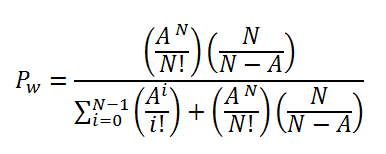# Erlang C Formula

Posted in Operations and Supply Chain Terms, Total Reads: 3823

## Definition: Erlang C Formula

The Erlang C formula calculates the probability that a request has to wait due to the resources or servers being busy. Hence, this probability is sometimes called as Waiting Probability.

The formula can be given as,where,

PW = waiting probability

A = total traffic offered in Erlangs

N = number of resources or servers

And the call arrivals follow a Poisson distribution.

The Erlang C formula is used to calculate the minimum number of servers of resources that would be required to achieve a required probability of service delivery during peak times with a limited probability of waiting for the requests.

Browse the definition and meaning of more terms similar to Erlang C Formula. The Management Dictionary covers over 7000 business concepts from 6 categories.

Search & Explore : Management Dictionary

Similar Definitions from same Category: